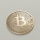Home
IT Knowledge
Inspiration
Languages
EN

# JavaScript - validate email with regex

20 points
Created by:Mikolaj
249

In this article, we would like to show how to validate e-mail address in JavaScript using regular expressions (`RegExp` class).

The first, most important thing is: there is no one way how to write an expression that validates an e-mail. Some solutions are more precise in checking correctness, other less.

Internet is filled with different solutions, so the main goal of the article is to gather all of them in one place.

Quick solution:

``````// ONLINE-RUNNER:browser;

var expression = /^[A-Z0-9._%+-]+@[A-Z0-9.-]+\.[A-Z]{2,}\$/i;

var email = 'john@gmail.com';
var result = expression.test(email);

console.log('e-mail is ' + (result ? 'correct' : 'incorrect'));``````

## AngualrJS expression example

Google in AngualrJS suggested its own expression that checks e-mail.

Note: full source code is located here.

Regular expression:

``/^(?=.{1,254}\$)(?=.{1,64}@)[-!#\$%&'*+/0-9=?A-Z^_`a-z{|}~]+(\.[-!#\$%&'*+/0-9=?A-Z^_`a-z{|}~]+)*@[A-Za-z0-9]([A-Za-z0-9-]{0,61}[A-Za-z0-9])?(\.[A-Za-z0-9]([A-Za-z0-9-]{0,61}[A-Za-z0-9])?)*\$/``

Working example:

``````// ONLINE-RUNNER:browser;

var expression = /^(?=.{1,254}\$)(?=.{1,64}@)[-!#\$%&'*+/0-9=?A-Z^_`a-z{|}~]+(\.[-!#\$%&'*+/0-9=?A-Z^_`a-z{|}~]+)*@[A-Za-z0-9]([A-Za-z0-9-]{0,61}[A-Za-z0-9])?(\.[A-Za-z0-9]([A-Za-z0-9-]{0,61}[A-Za-z0-9])?)*\$/;

var email = 'john@gmail.com';
var result = expression.test(email);

console.log('e-mail is ' + (result ? 'correct' : 'incorrect'));``````